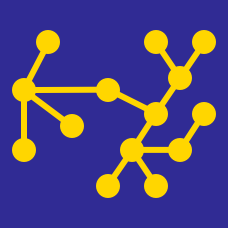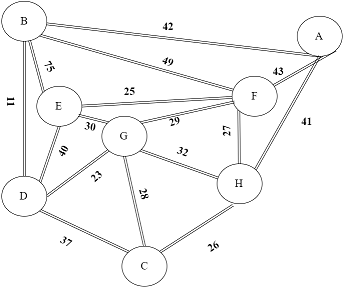Computer Science

# Spanning TreesWhat are the 3rd and 5th edges that Kruskal's algorithm includes for the graph above?

If we knew that all our input graphs have edges in the range $1$ to $K$(Where $K$ is a constant). If we use the most appropriate sorting algorithm, what is the most descriptive complexity of the overall run-time?

How many spanning trees does the following graph have?×